stat405

# ggplot2 drills

Recreate the graphs below by building them up layer by layer with ggplot2 commands.

## Airlines data set

1. Recreate the following plot of flight delays in Texas.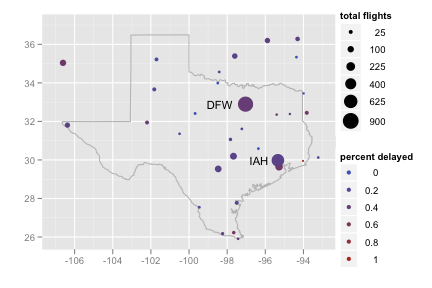Use
```library(maps) texas <- map_data("state", "texas")```
to retrieve the coordinates necessary to make a polygon in the shape of Texas.

```texas <- map_data("state", "texas")
texmap <- c(
geom_polygon(data = texas, colour = "grey70", fill = NA),
scale_x_continuous("", limits = c(-107, -93)),
scale_y_continuous("", limits = c(25.9, 37))
)

ggplot(feb13, aes(long, lat)) +
texmap +
geom_point(aes(size = ntot, colour = ndelay / ntot)) +
geom_text(aes(label = origin),
data = subset(feb13, ndelay >= 100),
size = 4, hjust = 1.5) +
scale_area("total flights", to = c(1, 8)) +
scale_colour_gradient("percent delayed")
```
2. Recreate the following plot of flights cancelled by size of the airport.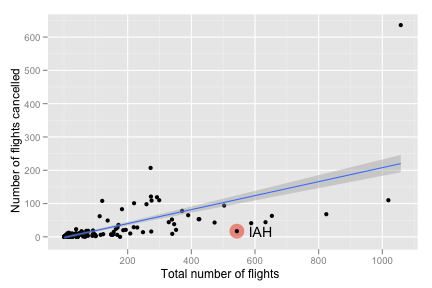```ggplot(feb13, aes(ntot, ncancel)) +
geom_point(data = subset(feb13, origin == "IAH"), size = 7,
colour = alpha("red", 0.5)) +
geom_point() +
geom_text(data = subset(feb13, origin == "IAH"),
aes(label = origin), hjust = -.5) +
geom_smooth(method = "lm", se = T) +
labs(y = "Number of flights cancelled",
x = "Total number of flights")
```
3. Recreate the following map of flight delays for airports with 100 or more flights on Feb. 13th.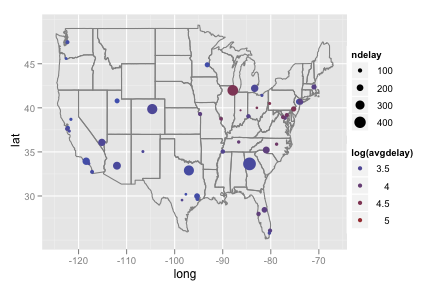```lower48 <- subset(feb13, long > -130)
lower48 <- subset(lower48, lat > 20)

ggplot(subset(lower48, ntot >= 100), aes(long, lat)) +
borders("state") +
geom_point(aes(size = ndelay, colour = log(avgdelay)))
```
4. Recreate the following plot of cancellations by longitude.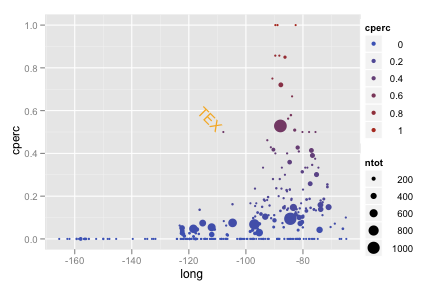```ggplot(feb13, aes(long, cperc)) +
geom_point(aes(colour = cperc, size = ntot)) +
geom_text(data = subset(feb13, cperc > 0.4 & long < -100),
aes(label = origin), hjust = 1.2, angle = -45,
colour = "orange")
```
5. Recreate the following plot of flight volume by longitude.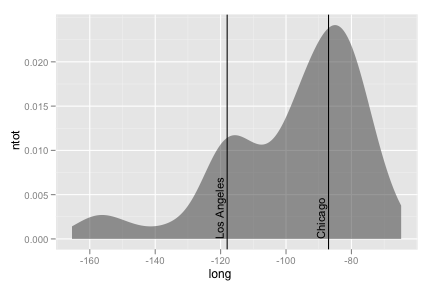Specify `stat = "density"` to generate the `..density.. variable.`

``` ggplot(feb13, aes(long, ntot)) + geom_area(aes(y = ..density..), stat = "density", alpha = 0.5) + geom_vline(xintercept = c(-118, -87)) + geom_text(aes(x,y, label = "Los Angeles"), data = data.frame(x = - 119, y = 0), size = 4, hjust = 0, vjust = 0, angle = 90) + geom_text(aes(x,y, label = "Chicago"), data = data.frame(x = -88, y = 0), size = 4, hjust = 0, vjust = 0, angle = 90) ```
6. ``` Recreate the following chart for the 11 busiest airports.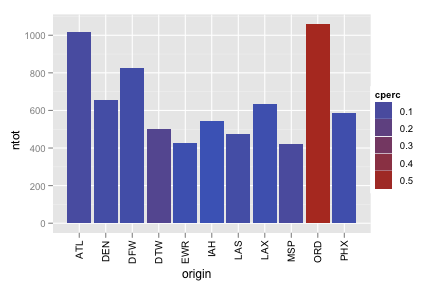Ensure you specified stringsAsFactors = F when you read in the data set. main <- subset(feb13, ntot > 400) ggplot(main, aes(origin, ntot)) + geom_bar(aes(fill = cperc)) + opts(axis.text.x = theme_text(angle = 90, hjust = 1)) Baby names data set Include the words that appear on the graph.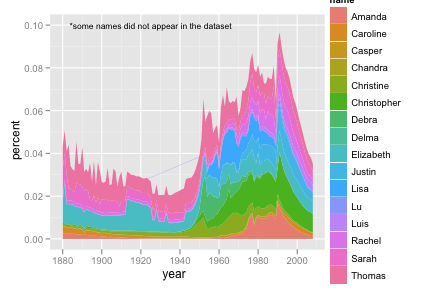Start by making the following data set names <- read.csv("baby-names.csv", header = T, stringsAsFactors = F) class <- c("Rakesh", "Luis", "Yanli", "Yen-yin", "Sarah", "Delma", "Chandra", "Elizabeth", "Kim-chi", "Amanda", "Thomas", "Caroline", "Da", "Christine", "Debra", "Christopher", "Justin", "Lisa", "Meng", "Emilian","Rachel", "Lu", "Casper", "Jingjing", "Chengyong", "Ruo", "Zhongyu") class_names <- subset(names, name %in% class) class_names <- ddply(class_names, c("name", "year"), summarise, percent = sum(percent) / length(percent)) ggplot(class_names, aes(year, percent)) + geom_area(aes(group = name, fill = name)) + geom_text(aes(year, percent, label = "*some names did not appear in the dataset"), data = data.frame(year = 1925, percent = 0.10), size = 4) Include the words that appear on the graph.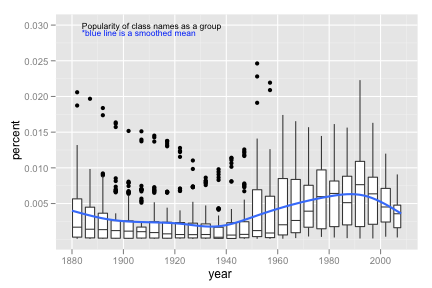Use the same data set as above Consider using round_any() to make continuous variables discrete. ggplot(class_names, aes(year, percent)) + geom_boxplot(aes(group = round_any(year, 5, floor))) + geom_smooth(se = F, size = 1) + geom_text(aes(year, percent, label = "*blue line is a smoothed mean"), colour = "blue", data = data.frame(year = 1906, percent = 0.029), size = 4) + geom_text(aes(year, percent, label = "Popularity of class names as a group"), data = data.frame(year = 1911, percent = 0.03), size = 4) Diamonds data set Create both graphs then comment on the merits of each one compared to the other.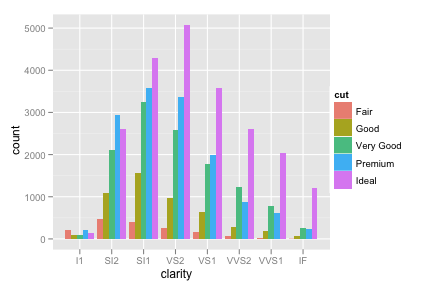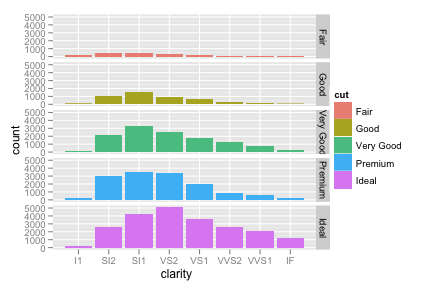ggplot(diamonds, aes(clarity)) + geom_bar(aes(fill = cut), position = "dodge") ggplot(diamonds, aes(clarity)) + geom_bar(aes(fill = cut)) + facet_grid(cut ~ .) Recreate the following pie chart.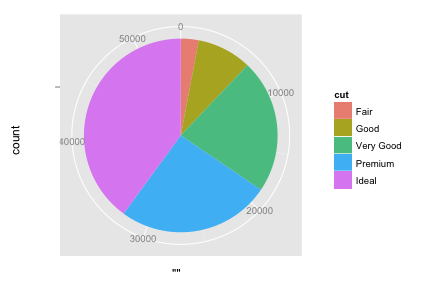To make a bar graph of the whole data set, specify aes(x = "", ...). What happens to the bar when you switch the x or y axis to polar coordinates? ggplot(diamonds, aes(x = "", fill = cut)) + geom_bar(width = 1) + coord_polar(theta = "y") Batting data set Recreate the following density map.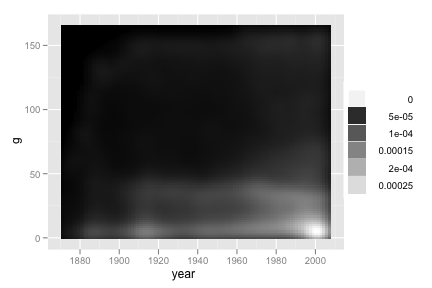Consider stat_density2d(..., contour = F). ggplot(b, aes(year, g)) + stat_density2d(geom = "tile", aes(fill = ..density..), contour = F) + scale_fill_gradient(low = "black", high = "white") Recreate the following line graph.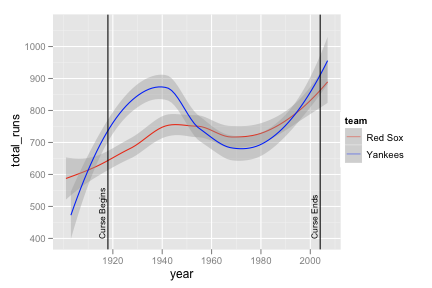yankees <- subset(b, team == "NYA") yankees <- transform(yankees, team = "Yankees") boston <- subset(b, team == "BOS") boston <- transform(boston, team = "Red Sox") yb <- rbind(yankees, boston) yb_runs <- ddply(yb, c("year", "team"), summarise, total_runs = sum(r, na.rm = T)) ggplot(yb_runs, aes(year, total_runs)) + geom_smooth(aes(colour = team)) + scale_colour_manual(value = c("red", "blue")) + geom_vline(aes(xintercept = c(1918, 2004))) + geom_text(aes(x,y, label = "Curse Begins"), data = data.frame(x = 1917, y = 400), size = 3, hjust = 0, vjust = 0, angle = 90) + geom_text(aes(x,y, label = "Curse Ends"), data = data.frame(x = 2003, y = 400), size = 3, hjust = 0, vjust = 0, angle = 90) Recreate the following line graph super imposed on the bar chart.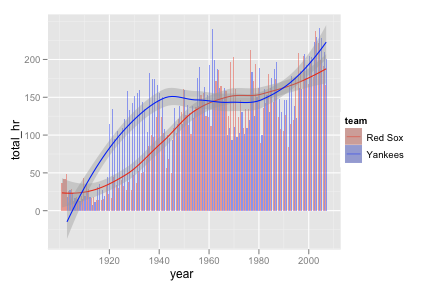yb_homeruns <- ddply(yb, c("year", "team"), summarise, total_hr = sum(hr, na.rm = T)) ggplot(yb_homeruns, aes(year, total_hr)) + geom_bar(aes(fill = team), stat = "identity", position = "dodge") + scale_fill_manual(value = alpha(c("red", "blue"), 0.4)) + geom_smooth(aes(colour = team)) + scale_colour_manual(value = c("red", "blue")) Recreate the following ovelapping areas graph.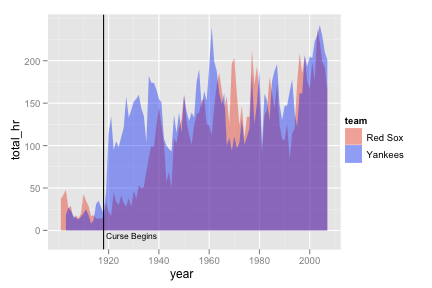Consider scale_fill_manual(). ggplot(yb_homeruns, aes(year, total_hr)) + geom_area(aes(fill = team), position = "identity") + scale_fill_manual(value = alpha(c("red", "blue"), 0.4)) + geom_vline(aes(xintercept = 1918)) + geom_text(aes(x,y, label = "Curse Begins"), data = data.frame(x = 1919, y = -10), size = 3, hjust = 0, vjust = 0) Recreate the following box plots.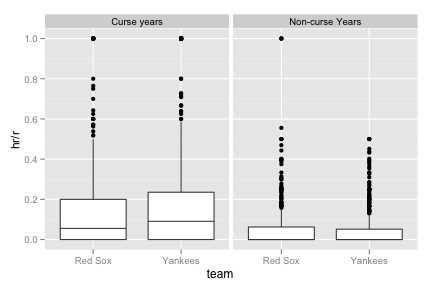yb_curse <- subset(yb, year > 1918 & year <= 2004) yb_curse <- transform(yb_curse, curse = "Curse years") yb_noncurse <- subset(yb, year <= 1918 | year > 2004) yb_noncurse <- transform(yb_noncurse, curse = "Non-curse Years") yb <- rbind(yb_curse, yb_noncurse) ggplot(yb, aes(team, hr / r)) + geom_boxplot() + facet_grid( . ~ curse) Players data set (or combined players/batting) Recreate the following map of countries baseball players have come from.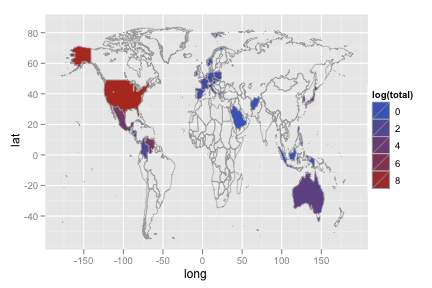library(maps) world_map <- map_data("world") names(world_map) <- "country" p_country <- ddply(p, "country", summarise, total = length(country)) p_map <- merge(p_country, world_map, by = "country", all = T) p_map <- p_map[order(p_map\$order), ] ggplot(p_map, aes(long, lat)) + geom_polygon(aes(group = group, fill = log(total)), colour = "grey60", size = .3) + ylim(-55, 85) Recreate the following chart of the 10 most represented foreign countries in the combined dataset.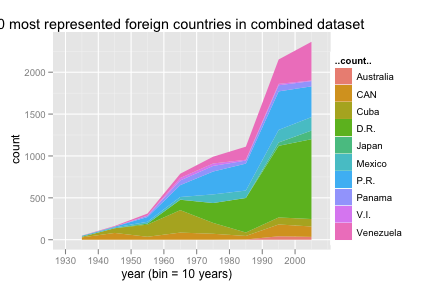Use stat = "bin" to generate the ..count.. variable. bp <- merge(b, p, by = "id") bp_country <- ddply(bp, "country", summarise, total = length(country)) bp_country <- bp_country[order(-bp_country\$total), ] bp_10 <- subset(bp, country %in% bp_country[2:11, 1]) ggplot(bp_10, aes(year)) + geom_area(aes(y = ..count.., fill = country), stat = "bin", binwidth = 10, position = "stack") + opts(title = "10 most represented foreign countries in combined dataset") + xlab("year (bin = 10 years)") Recreate the side by side bar charts.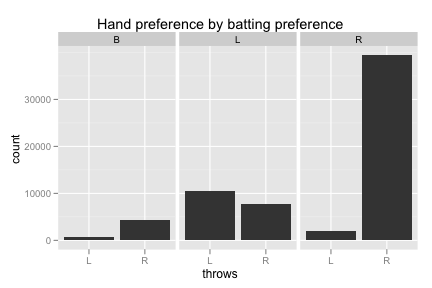bp_trimmed <- subset(bp, bats != "") ggplot(bp_trimmed, aes(throws)) + geom_bar() + facet_grid (. ~ bats) + opts(title = "Hand preference by batting preference") Recreate the following scatterplot.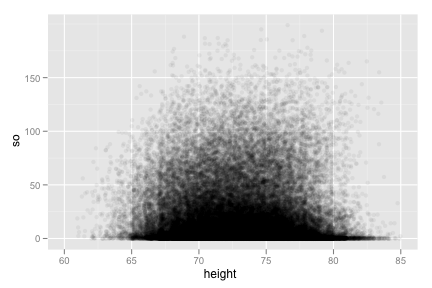ggplot(bp, aes(height, so)) + geom_jitter(position = position_jitter(width = 5), alpha = 0.05) + xlim(60, 85) Recreate the following comparison of players who have hit more than 60 homeruns in a season.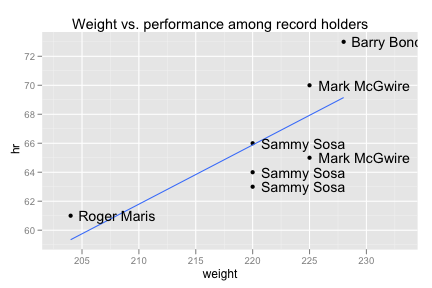ggplot(subset(bp, hr > 60), aes(weight, hr)) + geom_point() + geom_smooth(method = "lm", se = F) + geom_text(aes(label = paste(first, last, sep = " ")), hjust = -0.1) + xlim(203, 233) + opts(title = "Weight vs. performance among record holders") ```
``` ```
``` ```
``` Styled with skeleton and subtlepatterns. Built with jekyll. Hosted on S3. ```
``` ```